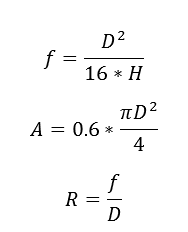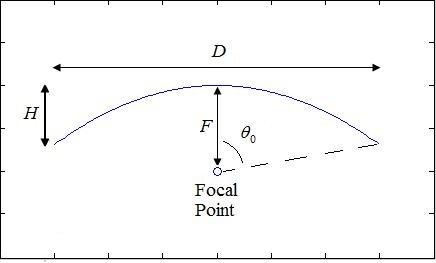# Parabolic Dish Antenna Calculator

This CalcTown calculator calculates the focal length, ratio and effective aperture of a parabolic dish antenna.

m
m

#### Result

m
m2Click here to view image

Where,

D = Antenna diameter length

H = Antenna dish depth

f = Focal length of the Antenna

R = Focal ratio of the antenna

A = Effective aperture of the Antenna